Varsity Tutors always has a different Calculus 2 Question of the Day ready at your disposal! If you’re just looking to get a quick review into your busy day, our Calculus 2 Question of the Day is the perfect option. Answer enough of our Calculus 2 Question of the Day problems and you’ll be ready to ace the next test. Check out what today’s Calculus 2 Question of the Day is below.

### Question of the Day: Calculus 2

Define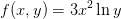.

Find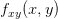.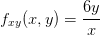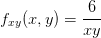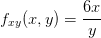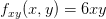Calculus II courses delve further into the ideas of limits, integrals, and derivatives introduced in detail in Calculus I courses. For this reason, Calculus II courses usually enforce prerequisite courses of Calculus I and previous math classes. Calculus II concepts are applied in Calculus III, Differential Equations, and other higher-level mathematics courses, as well as in the sciences. Whether you need Calculus tutoring in AtlantaCalculus tutoring in Houston, or Calculus tutoring in San Francisco, working one-on-one with an expert may be just the boost your studies need.

Like Calculus I courses, Calculus II courses discuss limits, stressing the concept of a limit, the association between limits and asymptotes along with limits and continuity, as well as finding limits and one-sided limits. Calculus II also introduces the concepts of parametric, polar, and vector equations. When discussing parametric equations, Calculus II classes teach parametric calculations, graphing parametrics, derivatives of parametrics, and parametric form. When talking about polar equations, Calculus II courses introduce the concepts of polar calculations and polar form, as well as graphing polar form and derivatives of polar form. Vectors are also taught in detail, with classes helping students to learn about vector form, vector calculations, graphing vectors, and derivatives of vectors.

Derivatives and integrals also form a fundamental part of Calculus II courses as students learn more about these crucial Calculus I concepts. The idea of a derivative, how to find a derivative at a point, and first and second derivatives of functions are all concepts discussed in Calculus II that should be review from Calculus I courses. New concepts on the topic of derivatives taught in Calculus II include L’Hospital’s Rule and Euler’s Method. Integrals are similarly reviewed, with particular attention paid to the definition of an integral, the Fundamental Theorem of Calculus, and Riemann sums before new concepts of defining integrals, solving integrals by substitution, improper integrals, and indefinite integrals are introduced. Students are taught to apply their knowledge of integrals in the contexts of finding the volume of a solid, the average values and lengths of functions, the area under a curve, and various other applications derived from physics. Varsity Tutors offers resources like free Calculus II Practice Tests to help with your self-paced study, or you may want to consider an Calculus II tutor.

The major topic that Calculus II courses introduce that is not taught in Calculus I is series. After series and functions are introduced to students, the concepts of convergence and divergence are taught, including comparing series and the ratio test. Students become familiar with various kinds of series, including alternating series, harmonic series, arithmetic series, and geometric series. Students are also taught about Taylor series, Maclaurin series, and Power series, discussing the concepts of Lagrange error in the process. In addition to the Calculus II Question of the Day and Calculus II tutoring, you may also want to consider taking some of our Calculus II Flashcards.

Being successful in Calculus II requires one to have a solid Calculus I background on which to base the new knowledge which the course introduces. If you find that your Calculus knowledge is a bit shaky and it is difficult to understand the new concepts that are introduced in your Calculus II course, practicing more Calculus II problems may be a solution to help you improve your Calculus understanding. Varsity Tutors’ free Calculus II Practice Tests are always available online for free for you to practice Calculus II concepts as much as you need to in order to understand them. Each Practice Test consists of ten to twelve Calculus problems, and each one comes with a complete explanation of how to find the correct answer. Problems are organized in Practice Tests, which draw from a variety of topics, and by concepts, allowing you to work exclusively on whichever particular topics are giving you trouble. Using Varsity Tutors’ variety of Calculus II resources, you can work at your own pace and focus on the concepts that you understand least well in order to master Calculus II in an efficient and thorough manner.

### All AP Calculus Resources